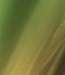## Presenting several pictures in a randomized order with an input box beneath each (6 pics, 6 boxes)

##### Presenting several pictures in a randomized order with an input box...
 Author Message AlmutusGroup: Forum Members Posts: 3, Visits: 11 Hi!I'm trying to create a "page" that shows 6 pictures in a randomized order for a serial order task - so underneath each picture should be a box, where one can write (or choose) the numbers 1 through 6 to indicate the correct order of the pictures. Now, I've been sucessfull in creating a page with the randomized pics, but I cannot put more than one input box beneath it. I was trying it with an openended element, since survey-pages cannot show pictures (,if I'm right).Does anyone have an idea, how to do this?Thank You!Almutus Daveposted 9 Years Ago ANSWERGroup: Administrators Posts: 12K, Visits: 93K isn't going to work for this -- it can't have multiple input boxes. / is indeed the way to go -- and you *can* in fact display elements on a via its /stimulusframes attribute. AlmutusGroup: Forum Members Posts: 3, Visits: 11 Thanks! This worked out! AlmutusGroup: Forum Members Posts: 3, Visits: 11 Hello!I've come across another problem now: I've already written that I randomized the presentation order of the pictures and underneath each I've put a textbos, where subject are supposed to indicate the correct order. This works fine now, but it doesn't record, where it positioned which picture, so I cannot put together the response of the subjects with the actual position of the pictures on the surveypage. Can I do that somehow with an "ontrialbegin"?Here's my script:/ fontstyle = ("Verdana", 2%, true)/ trials = [1=Position_Test]+++++++++++++++++++++++++++++++++++++++++++++++++++++++++++++ surveypage presents 6 stimuli /w 6 boxes+++++++++++++++++++++++++++++++++++++++++++++++++++++++++++++/ stimulusframes = [1=pic1_sim_L1,pic2_sim_L1,pic3_sim_L1,pic4_sim_L1,pic5_sim_L1,pic6_sim_L1]/ questions = [1=Position1, Position2, Position3, Position4, Position5,Position6]/ finishlabel = ("      weiter      ")/ subcaption = ("Geben Sie die richtige Position der Bilder an, indem Sie die entsprechende Ziffern (1-6) in die Kästchen schreiben.")+++++++++++++++++++++++++++++++++++++++++++++++++++++++++++++Pictures_vertical position constant+++++++++++++++++++++++++++++++++++++++++++++++++++++++++++++***** pics visually similar list 1 *****/ select = noreplace/ items = ("Apfel.bmp")/size = (15%,15%)/ vposition = 45%/ hposition = counter.picpos.selectedvalue/ select = noreplace/ items = ("Rad.bmp")/size = (15%,15%)/ vposition = 45%/ hposition = counter.picpos.selectedvalue/ select = noreplace/ items = ("Bombe.bmp")/size = (15%,15%)/ vposition = 45%/ hposition = counter.picpos.selectedvalue/ select = noreplace/ items = ("Knopf.bmp")/size = (15%,15%)/ vposition = 45%/ hposition = counter.picpos.selectedvalue/ select = noreplace/ items = ("Ball.bmp")/size = (15%,15%)/ vposition = 45%/ hposition = counter.picpos.selectedvalue/ select = noreplace/ items = ("Teller.bmp")/size = (15%,15%)/ vposition = 45%/ hposition = counter.picpos.selectedvalue+++++++++++++++++++++++++++++++++++++++++++++++++++++++++++++ Candidate positions for pics+++++++++++++++++++++++++++++++++++++++++++++++++++++++++++++/ items = (10%,25%,40%,55%,70%,85%)/ select = noreplace/ selectionrate = always+++++++++++++++++++++++++++++++++++++++++++++++++++++++++++++/ mask = positiveinteger/ range = (1, 6)/ position = (9%,55%)/ textboxsize = (2,3)/ mask = positiveinteger/ range = (1, 6)/ position = (24%,55%)/ textboxsize = (2,3)/ mask = positiveinteger/ range = (1, 6)/ position = (39%,55%)/ textboxsize = (2,3)/ mask = positiveinteger/ range = (1, 6)/ position = (54%,55%)/ textboxsize = (2,3)/ mask = positiveinteger/ range = (1, 6)/ position = (69%,55%)/ textboxsize = (2,3)/ mask = positiveinteger/ range = (1, 6)/ position = (84%,55%)/ textboxsize = (2,3) Daveposted 9 Years Ago ANSWERGroup: Administrators Posts: 12K, Visits: 93K Yes. Set up a bunch of (one for each pictures horizontal position)/ pic1_hpos = 0/ pic2_hpos = 0...use those in the 's /hposition attributes.../ hposition = values.pic1_hpospopulate them /ontrialbegin/ ontrialbegin = [values.pic1_hpos=counter.picpos.selectedvalue; values.pic2_hpos=counter.picpos.selectedvalue; ...]...and optionally log them to the data file/ columns = [..., values.pic1_hpos, values.pic2_hpos, ...]... Edited 9 Years Ago by Dave

#### Merge Selected

Merge into selected topic...

Merge into merge target...

Merge into a specific topic ID...